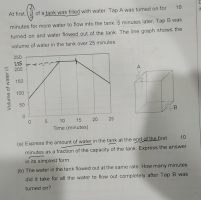# I am in P6;this year I am stuck with this question any kind one here help me to understand thk youp

#### Subhotosh Khan

##### Super Moderator
Staff member
What was the volume of water (Liters) in the tank at the begining (t = 0)?

If that is 1/5 volume of the tank - how much water can the tank hold when it is filled?

continue.....

Please show us what you have tried and exactly where you are stuck.

Please follow the rules of posting in this forum, as enunciated at:#### JeffM

##### Elite Member
This is a bad question. The implication is that you can tell from the graph what the relevant numbers are exactly. This is not true. The best that you can do is estimate the numbers from the graph. Graphs do not replace numbers, rather graphs supplement our understanding of numbers.

Nevertheless, you will be asked inept questions all your life so do your best to answer the question by estimating what the graph says the relevant numbers are and writing them down so you can think. At that point, this becomes just an arithmetic problem. (It can also be treated as a very simple algebra problem, but I am guessing that you have not learned any algebra yet.)

#### JustYourAverageFriend

##### New member
At the start the tank was 1/5 filled right? So, according to the graph that would be around 75L. The whole tank’s capacity would be 375L. At the end of 10 minutes, there was 225L, so the answer to (a) would be 225/375 simplified equals 9/15

For (b), looking at the graph, 50L of water was lost every 5 minutes, so calculate that to 10L per minute.
There was 225L in the tank so it would take 22.5minutes for all the water to flow out. Or 22 minutes and 30 seconds

#### JustYourAverageFriend

##### New member
What was the volume of water (Liters) in the tank at the begining (t = 0)?

If that is 1/5 volume of the tank - how much water can the tank hold when it is filled?

continue.....

Please show us what you have tried and exactly where you are stuck.

Please follow the rules of posting in this forum, as enunciated at:

View attachment 28197
If you look at the graph, it shows at 0 minutes there was 75L of water.

#### JeffM

##### Elite Member
At the start the tank was 1/5 filled right? So, according to the graph that would be around 75L. The whole tank’s capacity would be 375L. At the end of 10 minutes, there was 225L, so the answer to (a) would be 225/375 simplified equals 9/15

For (b), looking at the graph, 50L of water was lost every 5 minutes, so calculate that to 10L per minute.
There was 225L in the tank so it would take 22.5minutes for all the water to flow out. Or 22 minutes and 30 seconds
Looks good to me.

#### lex

##### Full Member
Nice work. Don't forget to cancel the fraction [MATH]\dfrac{9}{15}[/MATH] to [MATH]\dfrac{3}{5}[/MATH]
(Notice, out of interest, 75 litres at the start is [MATH]\dfrac{1}{5}[/MATH], so [MATH]3 \times 75[/MATH] litres = 225 litres is [MATH]\dfrac{3}{5}[/MATH]).